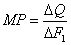Introduction to Economics

# What Is Law of Diminishing Returns In Economics

The law of diminishing returns (or, technically, diminishing marginal returns) is defined this way: If units of a resource are added to a fixed proportion of other resources, eventually marginal output will decline.A British economist, David Ricardo, found  the law of diminishing return in economy.

Example of law of diminishing returns.

Someone cultivates carrots on the land. The use of fertilizer is one of the inputs that will certainly support the success of the carrot crop.However,  Fertilizer should be used in accordance with the proportion needed. If the fertilizer  is used proportionally then the crop will be good. Conversely, if the fertilizer is added continuously it will cause adverse effects on the growth, so that the harvest of carrots will decline.

The Law of Diminishing Return is a law in economics that explains the proper proportion of inputs to get maximum output.This theory explains that when the input exceeds the production capacity , then our  return (income) will decrease. There are three levels in this theory, namely the phase of  increasing return,the second phase in which fixed income increases but at a lower intensity and the third phase is diminishing returns.

## The Assumption of Law of Diminishing Returns.:

• Only 1 variable changed with the other fixed.
• The technology used in the production process has not changed.
• The nature of the coefficient of production is variable.

Productivity Marginal

Marginal productivity is a factor increase in production resulting from use of another unit of the input while keeping constant the amounts used of other factors. Marginal productivity of capital = Increase in output / Increase in one unit of capital.

Production opportunities are the opportunities for the society to produce economic goods with full and effective use of all available resources at a given level of technology development. Possible output is characterized by a production capacity curve.

Suppose that F1 is a variable factor, while the other factors are constant.

The aggregate product ( Q or TP) is the amount of economic benefit produced using a certain amount of variable factor. Dividing the cumulative product by the amount of the variable factor consumed, we obtain the average product (AP).

The marginal product ( MP ) is defined as the increment of the aggregate product obtained as a result of infinitesimal increments in the amount of the variable factor used:For the measurement of labor productivity, the concept of average productivity or production (APL) and marginal productivity or output (MPL) is used:where TPL is the total labor productivity.

The law of diminishing marginal productivity (diminishing returns) asserts that with the growth of the use of any production factor (with the invariability of the rest), sooner or later a point is reached in which the additional application of the variable factor leads to a decrease in the relative and further absolute volumes of output.

The law of diminishing productivity has never been proven strictly theoretically, it is derived experimentally.First, it is applicable in the short run. Secondly, technological progress constantly pushes its boundaries. The first portions of labor, attached to a given amount of capital, ensure the growth of output, outrunning the growth of the amount of labor involved in production. This continues until a technologically optimal balance of labor and capital is reached. Further, output growth begins to lag behind the growth in the amount of labor employed.

Factors of production are used in production only when their productivity is a positive value. If we designate the marginal product in monetary terms through the MRP, and the marginal cost through the MRC, then the resource usage rule can be expressed by:

MRP = MRC